# 从Excel到Python：最常用的36个Pandas函数

Excel Python Pandas 时间：2019-12-03  查看：6   收藏

## 生成数据表Python支持从多种类型的数据导入。在开始使用Python进行数据

```import numpy as np
import pandas as pd```

```df=pd.DataFrame(pd.read_csv('name.csv',header=1))

```df = pd.DataFrame({"id":[1001,1002,1003,1004,1005,1006],
"date":pd.date_range('20130102', periods=6),
"city":['Beijing ', 'SH', ' guangzhou ', 'Shen
zhen', 'shanghai', 'BEIJING '],
"age":[23,44,54,32,34,32],
"category":['100-A','100-B','110-A','110-C','2
10-A','130-F'],
"price":[1200,np.nan,2133,5433,np.nan,4432]},
columns =['id','date','city','category','age',
'price'])```## 数据表检查

1.数据维度(行列)

Excel中可以通过CTRL+向下的光标键，和CTRL+向右的光标键

`df.shape`

2.数据表信息

# 数据表信息

```df.info()
<class 'pandas.core.frame.DataFrame'>
RangeIndex: 6 entries, 0 to 5
Data columns (total 6 columns):
id 6 non-null int64
date 6 non-null datetime64[ns]
city 6 non-null object
category 6 non-null object
age 6 non-null int64
price 4 non-null float64
dtypes: datetime64[ns](1), float64(1), int64(2), object(2)
memory usage: 368.0+ bytes```

3.查看数据格式

Excel中通过选中单元格并查看开始菜单中的数值类型来判断数Dtypes是一个查看数据格式的函数，可以一次性查看数据表中所

```#查看数据表各列格式
df.dtypes
id int64
date datetime64[ns]
city object
category object
age int64
price float64
dtype: object
#查看单列格式
df['B'].dtype
dtype('int64')```

4.查看空值

Excel中查看空值的方法是使用“定位条件”在“开始”目录下的“查找和选择”目录.Isnull是Python中检验空值的函数

```#检查数据空值
df.isnull()``````#检查特定列空值
df['price'].isnull()```5.查看唯一值

Excel中查看唯一值的方法是使用“条件格式”对唯一值进行颜色Python中使用unique函数查看唯一值。

```#查看city列中的唯一值
df['city'].unique()
array(['Beijing ', 'SH', ' guangzhou ', 'Shenzhen', 'shanghai', '
BEIJING '], dtype=object)```

6.查看数据表数值

Python中的Values函数用来查看数据表中的数值

```#查看数据表的值
df.values```7.查看列名称

Colums函数用来单独查看数据表中的列名称。

```#查看列名称
df.columns
Index(['id', 'date', 'city', 'category', 'age', 'price'], dtype='
object')```

8.查看前10行数据

```#查看前3行数据

9.查看后10行数据

```#查看最后3行
df.tail(3)```

## 数据表清洗

1.处理空值(删除或填充)

Excel中可以通过“查找和替换”功能对空值进行处理Python中处理空值的方法比较灵活，可以使用 Dropna函数用来删除数据表中包含空值的数据，也可以使用fillna函数对空值进行填充。

```#删除数据表中含有空值的行
df.dropna(how='any')``````#使用数字0填充数据表中空值
df.fillna(value=0)```

```#使用price均值对NA进行填充
df['price'].fillna(df['price'].mean())
Out:
0    1200.0
1    3299.5
2    2133.0
3    5433.0
4    3299.5
5    4432.0
Name: price, dtype: float64```2.清理空格

```#清除city字段中的字符空格
df['city']=df['city'].map(str.strip)```

3.大小写转换

Excel中有UPPER，LOWER等函数，Python中也有同名函数用来解决

```#city列大小写转换
df['city']=df['city'].str.lower()```4.更改数据格式

Excel中通过“设置单元格格式”功能可以修改数据格式。Python中通过astype函数用来修改数据格式。

```#更改数据格式
df['price'].astype('int')
0 1200
1 3299
2 2133
3 5433
4 3299
5 4432
Name: price, dtype: int32```

5.更改列名称

Rename是更改列名称的函数，我们将来数据表中的category列更改为category-size。

```#更改列名称
df.rename(columns={'category': 'category-size'})```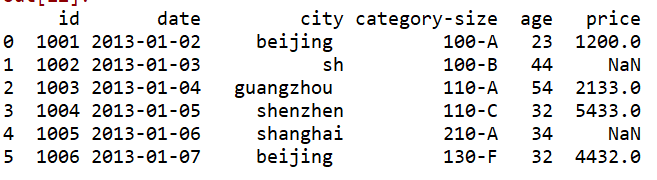6.删除重复值

Excel的数据目录下有“删除重复项”的功能Python中使用drop_duplicates函数删除重复值

```df['city']
0 beijing
1 sh
2 guangzhou
3 shenzhen
4 shanghai
5 beijing
Name: city, dtype: object```

city列中beijing存在重复，分别在第一位和最后一位
drop_duplicates()函数删除重复值

```#删除后出现的重复值
df['city'].drop_duplicates()
0 beijing
1 sh
2 guangzhou
3 shenzhen
4 shanghai
Name: city, dtype: object```

```#删除先出现的重复值
df['city'].drop_duplicates(keep='last')
1 sh
2 guangzhou
3 shenzhen
4 shanghai
5 beijing
Name: city, dtype: objec```

7.数值修改及替换

Excel中使用“查找和替换”功能就可以实现数值的替换Python中使用replace函数实现数据替换

```#数据替换
df['city'].replace('sh', 'shanghai')
0 beijing
1 shanghai
2 guangzhou
3 shenzhen
4 shanghai
5 beijing
Name: city, dtype: object```

## 数据预处理

1.数据表合并

```#建立df1数据表
df1=pd.DataFrame({"id":[1001,1002,1003,1004,1005,1006,1007,1008],
"gender":['male','female','male','female','male
','female','male','female'],
"pay":['Y','N','Y','Y','N','Y','N','Y',],
"m-point":[10,12,20,40,40,40,30,20]})```df_inner。

```#数据表匹配合并
df_inner=pd.merge(df,df1,how='inner')``````df_left=pd.merge(df,df1,how='left')
df_right=pd.merge(df,df1,how='right')
df_outer=pd.merge(df,df1,how='outer')```

2.设置索引列

```#设置索引列
df_inner.set_index('id')```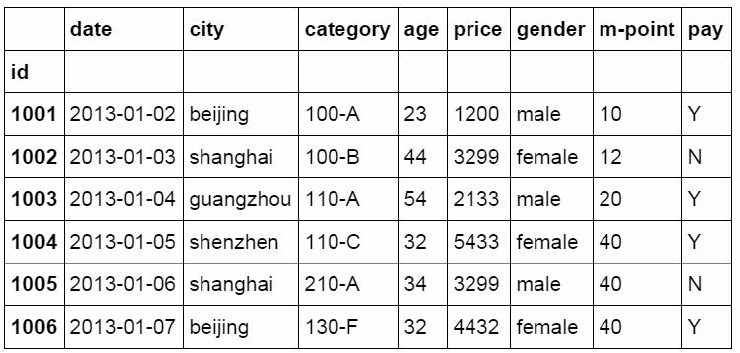3.排序(按索引，按数值)

Excel中可以通过数据目录下的排序按钮直接对数据表进行排Python中需要使用ort_values函数和sort_index函数完成排序

```#按特定列的值排序
df_inner.sort_values(by=['age'])```Sort_index函数用来将数据表按索引列的值进行排序。

```#按索引列排序
df_inner.sort_index()```4.数据分组

Excel中可以通过VLOOKUP函数进行近似匹配来完成对数值的分组，或者使用“数据透视表”来完成分组

Python中使用Where函数用来对数据进行判断和分组

```#如果price列的值>3000，group列显示high，否则显示low
df_inner['group'] = np.where(df_inner['price'] > 3000,'high','low
')```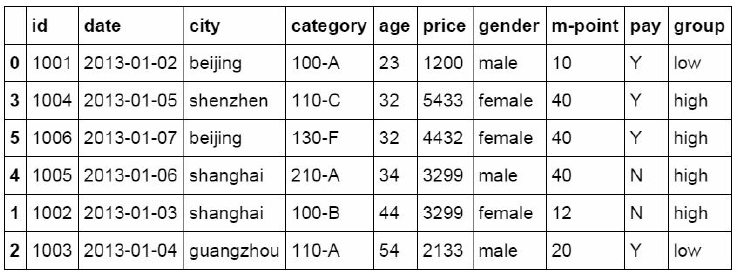```#对复合多个条件的数据进行分组标记
df_inner.loc[(df_inner['city'] == 'beijing') & (df_inner['price']
>= 4000), 'sign']=1```5.数据分列

Excel中的数据目录下提供“分列”功能。```#对category字段的值依次进行分列，并创建数据表，索引值为df_inner的索引列，列名称为category和size
pd.DataFrame((x.split('-') for x in df_inner['category']),index=d
f_inner.index,columns=['category','size'])``````#将完成分列后的数据表与原df_inner数据表进行匹配
df_inner=pd.merge(df_inner,split,right_index=True, left_index=Tru
e)```## 数据提取

1.按标签提取(loc)

```#按索引提取单行的数值
df_inner.loc
id 1004
date 2013-01-05 00:00:00
city shenzhen
category 110-C
age 32
price 5433
gender female
m-point 40
pay Y
group high
sign NaN
category_1 110
size C
Name: 3, dtype: object```

```#按索引提取区域行数值
df_inner.loc[0:5]```Reset_index函数用于恢复索引，这里我们重新将date字段的日期

```#重设索引
df_inner.reset_index()``````#设置日期为索引
df_inner=df_inner.set_index('date')``````#提取4日之前的所有数据
df_inner[:'2013-01-04']```2.按位置提取(iloc)

```#使用iloc按位置区域提取数据
df_inner.iloc[:3,:2]```iloc函数除了可以按区域提取数据，还可以按位置逐条提取

```#使用iloc按位置单独提取数据
df_inner.iloc[[0,2,5],[4,5]]```3.按标签和位置提取（ix）

ix是loc和iloc的混合，既能按索引标签提取，也能按位置进行数

```#使用ix按索引标签和位置混合提取数据
df_inner.ix[:'2013-01-03',:4]```4.按条件提取（区域和条件值）

```#判断city列的值是否为beijing
df_inner['city'].isin(['beijing'])
date
2013-01-02 True
2013-01-05 False
2013-01-07 True
2013-01-06 False
2013-01-03 False
2013-01-04 False
Name: city, dtype: bool```

```#先判断city列里是否包含beijing和shanghai，然后将复合条件的数据提取出来。
df_inner.loc[df_inner['city'].isin(['beijing','shanghai'])]```## 数据筛选

Excel数据目录下提供了“筛选”功能，用于对数据表按不同的条Python中使用loc函数配合筛选条件来完成筛选功能。配合sum和count函数还能实现Excel中sumif和countif函数的功能。

beijing。

```#使用“与”条件进行筛选
df_inner.loc[(df_inner['age'] > 25) & (df_inner['city'] == 'beiji
ng'), ['id','city','age','category','gender']]/```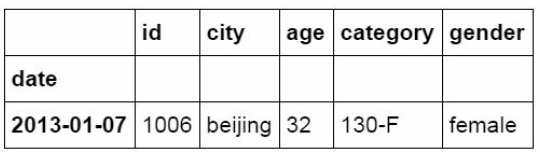```#使用“或”条件筛选
df_inner.loc[(df_inner['age'] > 25) | (df_inner['city'] == 'beiji
ng'), ['id','city','age','category','gender']].sort(['age'])``````#使用“非”条件进行筛选
df_inner.loc[(df_inner['city'] != 'beijing'), ['id','city','age',
'category','gender']].sort(['id'])``````#对筛选后的数据按city列进行计数
df_inner.loc[(df_inner['city'] != 'beijing'), ['id','city','age',
'category','gender']].sort(['id']).city.count()```

```#使用query函数进行筛选
df_inner.query('city == ["beijing", "shanghai"]')``````#对筛选后的结果按price进行求和
df_inner.query('city == ["beijing", "shanghai"]').price.sum()
12230```

## 数据汇总

Excel中使用分类汇总和数据透视可以按特定维度对数据进行汇总，Python中使用的主要函数是groupby和pivot_table。

1.分类汇总

```#对所有列进行计数汇总
df_inner.groupby('city').count()/``````#对特定的ID列进行计数汇总
df_inner.groupby('city')['id'].count()
city
beijing 2
guangzhou 1
shanghai 2
shenzhen 1
Name: id, dtype: int64```
```#对两个字段进行汇总计数
df_inner.groupby(['city','size'])['id'].count()
city size
beijing A 1
F 1
guangzhou A 1
shanghai A 1
B 1
shenzhen C 1
Name: id, dtype: int64```

```#对city字段进行汇总并计算price的合计和均值。
df_inner.groupby('city')['price'].agg([len,np.sum, np.mean])```2.数据透视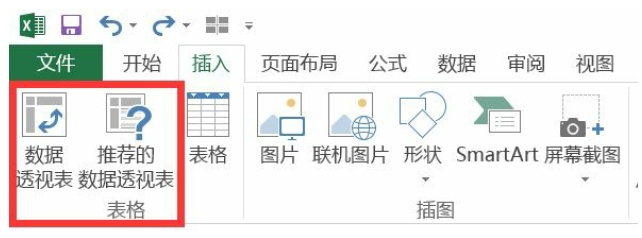Python中通过pivot_table函数实现同样的效果

```#设定city为行字段，size为列字段，price为值字段。

pd.pivot_table(df_inner,index=["city"],values=["price"],columns=[
"size"],aggfunc=[len,np.sum],fill_value=0,margins=True)```## 数据统计

1.数据采样

Excel的数据分析功能中提供了数据抽样的功能Python通过sample函数完成数据采样

```#简单的数据采样
df_inner.sample(n=3)```Weights参数是采样的权重，通过设置不同的权重可以更改采样的结果

```#手动设置采样权重
weights = [0, 0, 0, 0, 0.5, 0.5]
df_inner.sample(n=2, weights=weights)```Sample函数中参数replace，用来设置采样后是否放回

```#采样后不放回
df_inner.sample(n=6, replace=False)
#采样后放回
df_inner.sample(n=6, replace=True)```

2.描述统计

Python中可以通过Describe对数据进行描述统计

```#数据表描述性统计
df_inner.describe().round(2).T```3.相关分析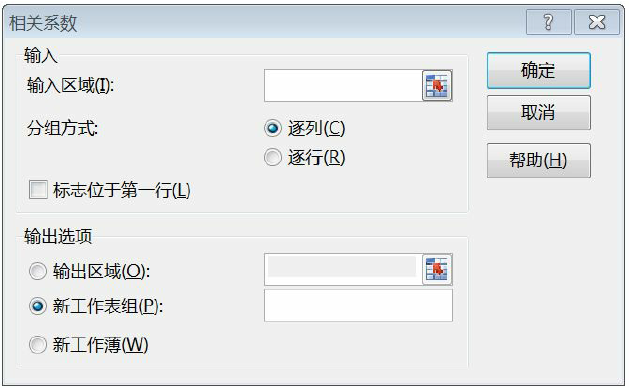Python中则通过corr函数完成相关分析的操作，并返回相关系数。

```#相关性分析
df_inner['price'].corr(df_inner['m-point'])
0.77466555617085264
#数据表相关性分析
df_inner.corr()```## 数据输出

1.写入Excel

```#输出到Excel格式
df_inner.to_Excel('Excel_to_Python.xlsx', sheet_name='bluewhale_c
c')```2.写入csv

```#输出到CSV格式
df_inner.to_csv('Excel_to_Python.csv')```

0% (0)
0% (0)